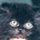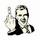## General Question# Desperate need of math homework help! (Merchandise Math)

Asked by ava (977) February 25th, 2008

I have been stuck on this one problem for hours…please help me through the steps to figuring out the problem. The answer is: 47.2% initial markup (I just need to know how to get there!)

The problem:
Determine the initial markup for a designer boutique that has the following projections for next season:

planned sales=\$4,000,000
Markdowns=\$600,000
Expenses=38%
Cash Discounts=4%
Shortages=\$20,000
Profit=5%

Formula: Initial MU%=Gross Margin%+Retail Reduction% divided by 100%+ Retail Reduction%

Observing members: 0Composing members: 0(9) Markup = The difference between the cost of an item and its selling price. This is the initial markup, or initial margin, before the impact of markdowns.

http://www.retail-consultant.com/retail_math

Retail Space Glossary of Retail Space Terms
http://www.retail—space.com/Retail-Space-Glossary-Of-Terms.htm

cool pdf presentation – check out page 19
http://www.smbtn.com/books/gb52.pdf

Marketing Calculators – This spreadsheet contains eight calculators you can use to make marketing decisions. It contains the following:
1) Sales Revenue – represents net revenue after sales returns and
allowances and discounts.
2) Cost of Goods Sold – the cost of the sales.
3) Gross Margin – Net revenue less cost of goods sold.
4) Selling Expense Ratio – Total selling expenses divided by sales.
5) Inventory Turnover – On average, the number of times per year you
“turnover” the inventory.
6) Mark-up based on Cost – Mark-up divided by cost.
7) Markup Based on Selling Price – Mark-up divided by selling price.
8) Markdown – Markdown in dollars divided by the original price.

Retail Sales Calculator
http://www.csgnetwork.com/retailsalescalc.html

Quick Books point of sale – VERY BIG FILE 712 PAGES

artemisdivine (1092)“Great Answer” (0) Flag as…or